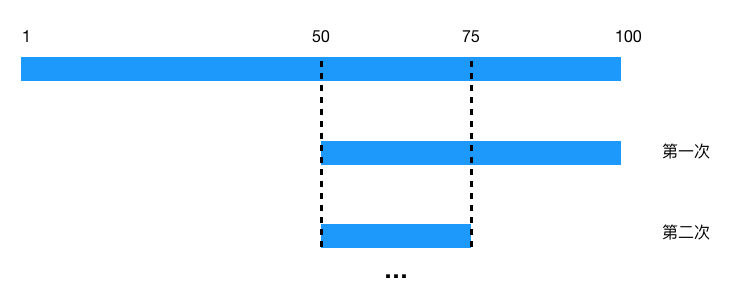# 优秀程序员必备的四项能力

danielmou

0收藏

### 前言

``````[202.102.133.0, 202.102.133.255]  山东东营市
[202.102.135.0, 202.102.136.255]  山东烟台
[202.102.156.34, 202.102.157.255] 山东青岛
[202.102.48.0, 202.102.48.255] 江苏宿迁
[202.102.49.15, 202.102.51.251] 江苏泰州
[202.102.56.0, 202.102.56.255] 江苏连云港``````### 抽象问题的能力

ip 地址不容易比较，那我们首先把 ip 地址转成整数，于是每个省市对应的 ip 地址区间就变成了整数区间,假设为如下区间

``````[1, 5]
[11, 15]
[16, 20]
[6, 10]
....``````

``````[1, 5]
[6, 10]
[11, 15]
[16, 20]
...``````

1. 首先猜 1 和 100 的中间数字(1+ 100) / 2 =  50（取整）
2. 50 < 70, 于是我们继续猜 50 和 100 的中间数字(50+100) / 2 = 75
3. 75 > 70，于是我们继续猜 50 和 75 的中间数字(50+75) / 2 = 62
4. 依次持续类似以上的步骤，不断地缩小范围，直至找到 70``````[1, 5]
[6, 10]
[11, 15]
[16, 20]
...``````

### 修改模型的能力

``````    public     static     int bsearch(    int[] a,     int length,     int{
int low = 0;
int high = length - 1;
while (low <= high) {
int mid = (low + high) / 2;
if (a[mid] > value) {
if (mid == 0) {
return -1;
}
if (a[mid-1] <= value) {
return mid-1;
}     else {
high = mid-1;
}
}    else {
low = mid + 1;
}
}
return -1;
}``````

### 代码要有足够的健壮性

``````    public     static     int bsearch(    int[] a,     int length,     int     throws{

if (length < 0) {
// 实际应该抛出一个继续自Exception的异常，这里为了方便直接抛出Exception
throw    new Exception("数据长度不合法");
}

int low = 0;
int high = length - 1;
while (low <= high) {
int mid = low + ((high - low) >> 1);
if (a[mid] > value) {
if (mid == 0) {
return -1;
}
if (a[mid-1] <= value) {
return mid-1;
}     else {
high = mid-1;
}
}    else {
low = mid + 1;
}
}
return -1;
}``````

### 代码要有足够的可扩展性

``````    public    static <T extends Comparable>     int bsearch(T[] a,     int     throws{
if (length < 0) {
// 实际应该抛出一个继承自Exception的异常，这里为了方便直接抛出Exception
throw    new Exception("数据长度不合法");
}
int low = 0;
int high = length - 1;
while (low <= high) {
int mid = low + ((high - low) >> 1);
if (a[mid].compareTo(value) > 0) {
if (mid == 0) {
return -1;
}
if (a[mid-1].compareTo(value) <= 0) {
return mid-1;
}     else {
high = mid-1;
}
}    else {
low = mid + 1;
}
}
return -1;
}``````

### 总结